The Half Adder Circuit operation needs two binary inputs : augend and addend bits; and two binary outputs : sum and carry. The half adder truth table shown in 3.6 gives the relation between input and output variables for Half Adder Circuit operation.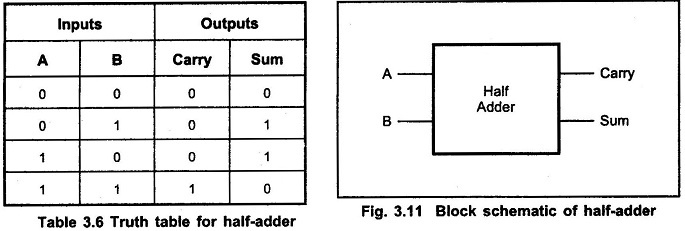### K-map simplification for carry and sum: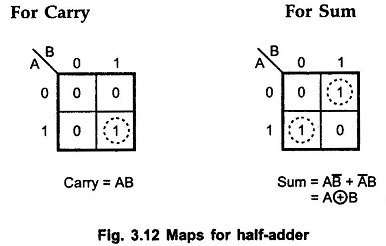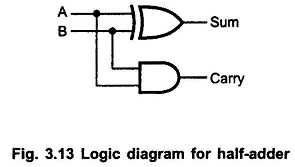We have seen that a full adder is a combinational circuit that forms the arithmetic sum of three input bits. It consists of three inputs and two outputs. Two of the input variables, denoted by A and B, represent the two significant bits to be added. The third input Cin, represents carry from the previous lower significant position.

The Full Adder Truth Table is shown in Table 3.7.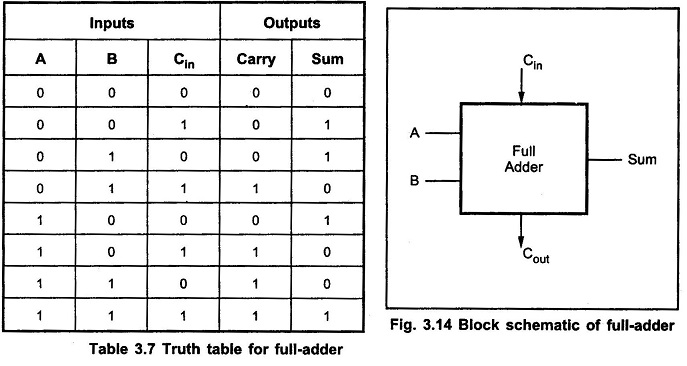### K-map simplification for carry and sum: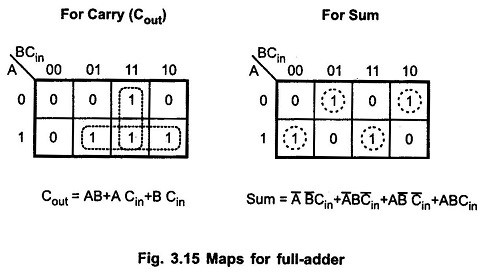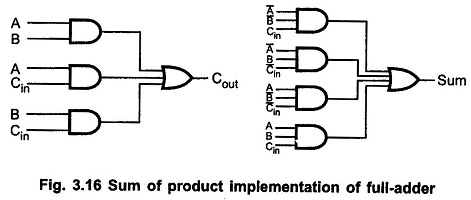The Boolean Expression for sum can be further simplified as follows :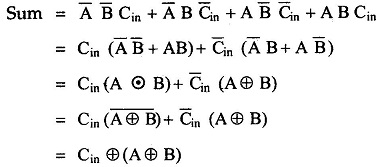With this simplified Boolean function circuit for full-adder can be implemented as shown in the Fig. 3.17.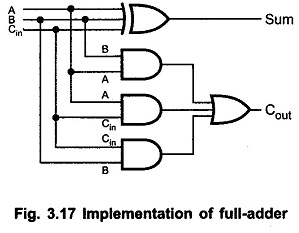A full-adder can also be implemented with two half-adders and one OR gate, as shown in the Fig. 3.18. The sum output from the second half-adder is the exclusive-OR of Cin and the output of the first half-adder, giving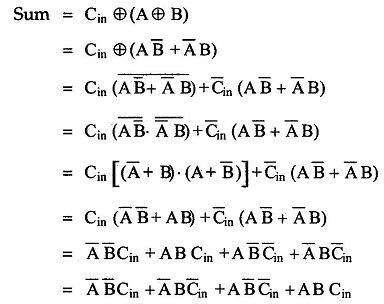and the carry output is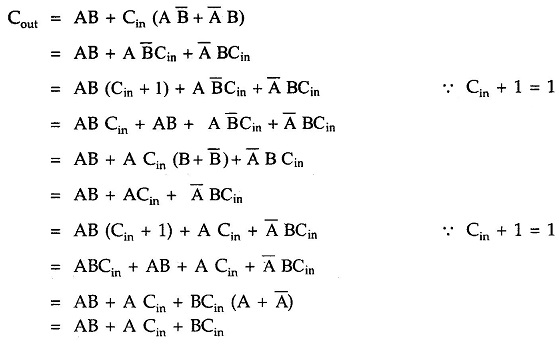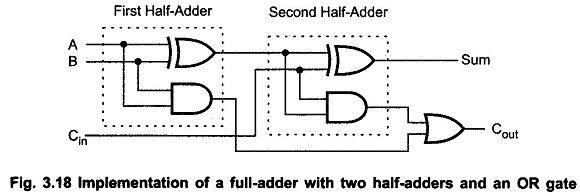Scroll to Top